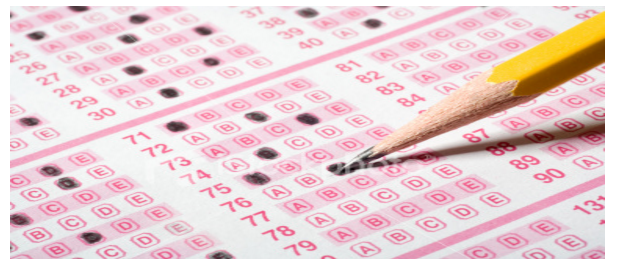# How to guess the right answer for JEE?Joint Entrance Examination (JEE) is an all India common engineering entrance examination, conducted by CBSE for admission in various engineering colleges all over the country. It is also regarded with one of the most challenging engineering admission tests internationally. JEE comprises of two parts i.e. JEE Mains and JEE Advanced.  JEE Advanced is for admission to Indian Institutes of Technology (IIT), while JEE-Main is for admission to Indian Institutes of Information Technology (IIIT) and National Institutes of Technology (NIT). Only the students who are selected in JEE Mains are eligible for appearing in JEE Advanced.

## JEE Exam Pattern

It’s a fact that the JEE exam is an objective type. In an exam, it’s imperative whether you understood the concept or not. All that matters is circling the correct bubble regardless of how you do it.

Negative marking is meant to discourage blind guessing. But if you are can guess sensibly, you may never receive any loss due to it. All the exams have four options for each question, and all the answers are equally distributed among the four choices i.e. a, b, c, and d which applies to all exams including IIT JEE. Therefore even in the worst case, if you mark all blind guesses, say option “a” the net difference in your score will be zero.

You don’t need to be surprised, because here’s the mathematical proof. Suppose you guessed in “x” number of questions and marked all of them as option “c.” By the law of probability, 75% of your guesses are wrong, and 25% are right. So your total is: [(X/4) x (+3)] – [(3X/4) x (-1)] = zero. Hence, there is zero addition to your score in the worst case.

### Tips And Tricks For JEE Mains

Let’s go through some tricks that will help IIT aspirants to clear JEE Mains.

• Highest to lowest

In a question wherein numerical value is the answer, the highest and lowest value will never be correct. This may seem weird, but it’s true for at least 60% of the cases. So, when you don’t know the right answer, stay away from extreme values. It might help you.

• Left with two options

If you have a doubt between two options, try to gamble on either. When you have managed to cross out the two options, go for one with the remaining two options. It works.

• No negatives

For questions which don’t carry negative marking, attempt them even if you have no idea about it. NEVER LEAVE THEM UNATTEMPTED. Since you have nothing to lose, and if you leave such questions, you will lose marks for no reason.

• Same and different

This applies to those questions which play in units and values.

Example 1: Units

Suppose the options for heat and thermodynamics question are:

(a) 140 C; (b) 130 C;  (c) 110 C; (d) 130 K

Since 3 of the options are given in degree Celsius, the answer will be most probably regarding degree Celsius. Next, since the value 130 is given in both Celsius and Kelvin, therefore 130 is the numerical answer. So, by the above logic, the answer will be the option (b) 130 C. Believe it or not, but this works for most of the cases.

Example 2: Mathematical extremes

Most of the times we find questions in Maths on either to find the domain or range of a function and the options are.

(a)    [(0,1) U (2,3) ; (b) (0,1) U (2,3)] ; (c) [0,1] U [2,3] ; (d) (0,1) U (2,3)

You don’t have to solve the whole function. Try to test the function on the extreme values i.e. 0, 1, 2, 3. Just see if these 4 values satisfy the function and you can get the best answer. It saves a lot of time.

• Value putting

This applies to questions where you need to find the general value for something. Suppose you have to find a value for the next number in a series. Take for example 2, 1, ½, ¼,…

What number should come next? (a)? (b)? (c)2/8 (d)1/16.

In these kinds of questions, look for the degree or direction of change between the values. Notice do the numbers increase or decrease, and by how much. For this question, it’s a simple division. Each no is one-half of the previous number. In other terms, the number is divided by 2 to get the next value.

Therefore, 4/2=2, 2/2=1, ½=½, (½)/2=¼, (¼)/2=? and so on. So option (b) is correct.

In case you want to test all these, take up an MCQ set of topics from BYJU’S. Attempt the above-mentioned tips on topics you have not studied and apply all the above logic and see your score. You will notice that these tricks have their own impact. Always remember that JEE tries to test your knowledge and understanding, but sometimes forgets to maintain logic in the options. To know more tips and tricks, please keep visiting BYJU’S.